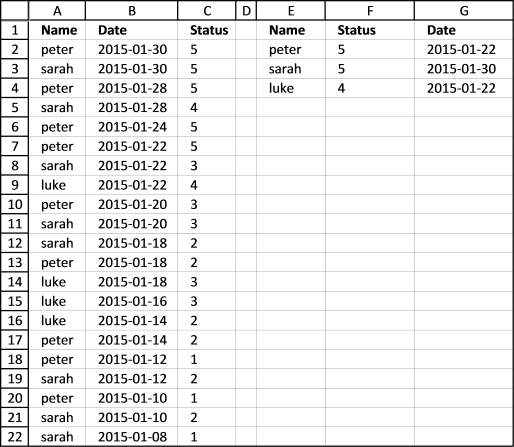Author: Oscar Cronquist Article last updated on July 15, 2017

Hello Oscar,
thank you for sharing you knowledge and helping us with these excellent formulas.
I have a case i could really need your help with:
The following Table shows a history of names, stati and the date the status was acquired. BUT: people can acquire the same status more than just once (or at least report it). Now I want to know, when each person (peter, sarah & luke) have acquired their individual highest status.

Name Date Status
peter 30.01.2015 5
sarah 30.01.2015 5
peter 28.01.2015 5
sarah 28.01.2015 4
peter 24.01.2015 5
peter 22.01.2015 5
sarah 22.01.2015 3
luke 22.01.2015 4
peter 20.01.2015 3
sarah 20.01.2015 3
sarah 18.01.2015 2
peter 18.01.2015 2
luke 18.01.2015 3
luke 16.01.2015 3
luke 14.01.2015 2
peter 14.01.2015 2
peter 12.01.2015 1
sarah 12.01.2015 2
peter 10.01.2015 1
sarah 10.01.2015 2
sarah 08.01.2015 1

Names latest status in status since
Peter 5 22.01.2015
sarah 5 30.01.2015
luke 4 22.01.2015

I would really appreciate your help.
Greetings.Array formula in F2:

=MAX(IF(E2=\$A\$2:\$A\$22, \$C\$2:\$C\$22, ""))

Array formula in cell G2:

=MIN(IF((MAX(IF(E2=\$A\$2:\$A\$22,\$C\$2:\$C\$22,""))=\$C\$2:\$C\$22)*(E2=\$A\$2:\$A\$22),\$B\$2:\$B\$22,"A"))

### How to enter an array formula

1. Select cell F2
2. Paste formula in formula bar
3. Press and hold CTRL + SHIFT simultaneously
4. Press Enter once
5. Release all keys

This is what the formula in the formula bar looks like:

{=MAX(IF(E2=\$A\$2:\$A\$22, \$C\$2:\$C\$22, ""))}

Don't enter these curly brackets yourself, they appear automatically when you press CTRL + SHIFT + ENTER.

### Explaining array formula in cell F2

Step 1 - Compare name in cell E2 with names in cell range A2:A22

E2=\$A\$2:\$A\$22

becomes

"peter"={"peter"; "sarah"; "peter"; "sarah"; "peter"; "peter"; "sarah"; "luke"; "peter"; "sarah"; "sarah"; "peter"; "luke"; "luke"; "luke"; "peter"; "peter"; "sarah"; "peter"; "sarah"; "sarah"}

becomes

{TRUE; FALSE; TRUE; FALSE; TRUE; TRUE; FALSE; FALSE; TRUE; FALSE; FALSE; TRUE; FALSE; FALSE; FALSE; TRUE; TRUE; FALSE; TRUE; FALSE; FALSE}

Step 2 - Replace TRUE with corresponding value in cell range C2:C22 and FALSE with nothing

IF(E2=\$A\$2:\$A\$22, \$C\$2:\$C\$22, "")

becomes

IF({TRUE; FALSE; TRUE; FALSE; TRUE; TRUE; FALSE; FALSE; TRUE; FALSE; FALSE; TRUE; FALSE; FALSE; FALSE; TRUE; TRUE; FALSE; TRUE; FALSE; FALSE}, \$C\$2:\$C\$22, "")

becomes

IF({TRUE; FALSE; TRUE; FALSE; TRUE; TRUE; FALSE; FALSE; TRUE; FALSE; FALSE; TRUE; FALSE; FALSE; FALSE; TRUE; TRUE; FALSE; TRUE; FALSE; FALSE}, {5; 5; 5; 4; 5; 5; 3; 4; 3; 3; 2; 2; 3; 3; 2; 2; 1; 2; 1; 2; 1}, "")

and returns

{5; ""; 5; ""; 5; 5; ""; ""; 3; ""; ""; 2; ""; ""; ""; 2; 1; ""; 1; ""; ""}

Step 3 - Find largest value in array

MAX(IF(E2=\$A\$2:\$A\$22,\$C\$2:\$C\$22,""))

becomes

=MAX({5; ""; 5; ""; 5; 5; ""; ""; 3; ""; ""; 2; ""; ""; ""; 2; 1; ""; 1; ""; ""})

and returns 5 in cell F2.

### Explaining array formula in cell G2

Step 1 - Compare largest value with cell range C2:C22

MAX(IF(E2=\$A\$2:\$A\$22,\$C\$2:\$C\$22,""))=\$C\$2:\$C\$22

becomes

5=\$C\$2:\$C\$22

and returns

{TRUE; TRUE; TRUE; FALSE; TRUE; TRUE; FALSE; FALSE; FALSE; FALSE; FALSE; FALSE; FALSE; FALSE; FALSE; FALSE; FALSE; FALSE; FALSE; FALSE; FALSE}

Step 2 - Compare value in cell E2 with cell range A2:A22

E2=\$A\$2:\$A\$22

returns

{TRUE; FALSE; TRUE; FALSE; TRUE; TRUE; FALSE; FALSE; TRUE; FALSE; FALSE; TRUE; FALSE; FALSE; FALSE; TRUE; TRUE; FALSE; TRUE; FALSE; FALSE}

Step 3 - Multiply array in step 1 with array in step 2

MAX(IF(E2=\$A\$2:\$A\$22,\$C\$2:\$C\$22,""))=\$C\$2:\$C\$22)*(E2=\$A\$2:\$A\$22)

becomes

{TRUE; TRUE; TRUE; FALSE; TRUE; TRUE; FALSE; FALSE; FALSE; FALSE; FALSE; FALSE; FALSE; FALSE; FALSE; FALSE; FALSE; FALSE; FALSE; FALSE; FALSE} * {TRUE; FALSE; TRUE; FALSE; TRUE; TRUE; FALSE; FALSE; TRUE; FALSE; FALSE; TRUE; FALSE; FALSE; FALSE; TRUE; TRUE; FALSE; TRUE; FALSE; FALSE}

and returns

{1; 0; 1; 0; 1; 1; 0; 0; 0; 0; 0; 0; 0; 0; 0; 0; 0; 0; 0; 0; 0}

Step 4 - Replace TRUE with corresponding value in cell range C2:C22 and FALSE with random text string

IF(E2=\$A\$2:\$A\$22,\$C\$2:\$C\$22,""))=\$C\$2:\$C\$22)*(E2=\$A\$2:\$A\$22),\$B\$2:\$B\$22,"A")

bcomes

IF({1; 0; 1; 0; 1; 1; 0; 0; 0; 0; 0; 0; 0; 0; 0; 0; 0; 0; 0; 0; 0},\$B\$2:\$B\$22,"A")

and returns

{42034; "A"; 42032; "A"; 42028; 42026; "A"; "A"; "A"; "A"; "A"; "A"; "A"; "A"; "A"; "A"; "A"; "A"; "A"; "A"; "A"}

Step 5 - Find smallest value in array, text strings are ignored

MIN(IF((MAX(IF(E2=\$A\$2:\$A\$22,\$C\$2:\$C\$22,""))=\$C\$2:\$C\$22)*(E2=\$A\$2:\$A\$22),\$B\$2:\$B\$22,"A"))

becomes

MIN({42034; "A"; 42032; "A"; 42028; 42026; "A"; "A"; "A"; "A"; "A"; "A"; "A"; "A"; "A"; "A"; "A"; "A"; "A"; "A"; "A"})

and returns 42026 in cell G2.